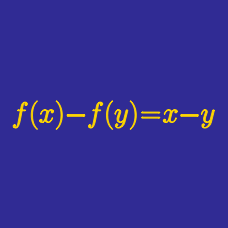Algebra

# Functional Equations - Problem Solving

The function $f$ satisfies $(x-y)f(x+y)-(x+y)f(x-y)+4xy(x^2-y^2)=0,$

whenever $|x| \neq |y |$.

Given that $f(1)=2,$ what is $f(x)$?

Function $f(n)$ defined over all positive integers $n$ satisfies the following: \begin{aligned} & f(1)=1, f(2)=2, \text{ and}\\ & f(n+2)=f(n+1)+\left(f(n)\right)^2+3581 \text{ for } n \geq 1. \end{aligned} How many of the $3581$ integers $f(1), f(2), \ldots, f(3581)$ are multiples of $7?$

What is the polynomial $p(x)$ that satisfies $p(x^2+4)=\left(p(x)\right)^2+4 \text{ and } p(0)=0?$

A function $f$ from the positive integers to the positive integers satisfies the conditions
1) $f(n) = 2 f(n-1) + 3$,
2) $f(1) = 9 .$

What is the value of $f(9)$?

If $f(x)$ is a polynomial satisfying $27 f(x^3) -4f(x^2) - x^6 f(3x) + 46 = 0$, what is $f(10)?$

×